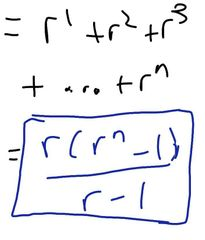• Shuffle
Toggle On
Toggle Off
• Alphabetize
Toggle On
Toggle Off
• Front First
Toggle On
Toggle Off
• Both Sides
Toggle On
Toggle Off
Toggle On
Toggle Off
Front

## Card Range To Study

throughPlay buttonPlay buttonProgress

1/11

Click to flip

Use LEFT and RIGHT arrow keys to navigate between flashcards;

Use UP and DOWN arrow keys to flip the card;

H to show hint;

 From 78a, you're given a sequence. How to obtain general term, an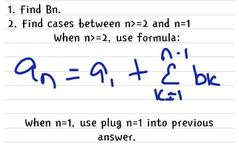Obtain sum of first n terms, Sn?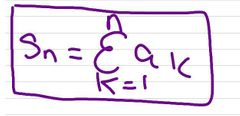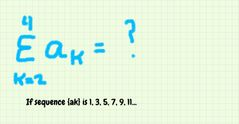N48a = a2 + a3 + a4 = 3 + 5 + 7 = 15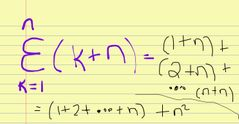N49a, Q6.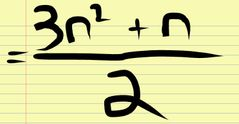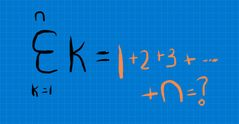N49a, Q5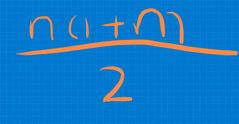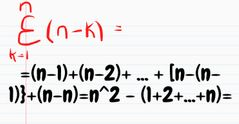N49a, Q8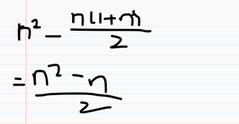Given the first term (a), common difference (d), and number of terms (n), which Sn formula do you use?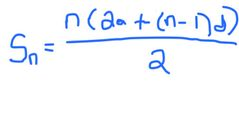Given the first term (a), last term (l), which Sn formula do you use?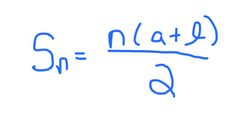If you are given common ratio (r) and first term (a), number of terms (n), which formula for Sn?R not = 1.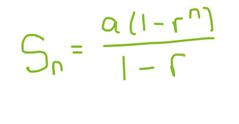If you are given common ratio (r) and first term (a), number of terms (n), which formula for Sn?R= 1.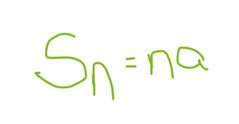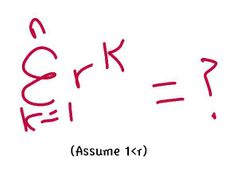N67b, Q5